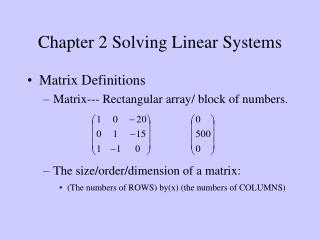DownloadDownload PresentationChapter 2 Solving Linear Systems

# Chapter 2 Solving Linear Systems

Download Presentation## Chapter 2 Solving Linear Systems

- - - - - - - - - - - - - - - - - - - - - - - - - - - E N D - - - - - - - - - - - - - - - - - - - - - - - - - - -
##### Presentation Transcript

1. Chapter 2 Solving Linear Systems • Matrix Definitions • Matrix--- Rectangular array/ block of numbers. • The size/order/dimension of a matrix: • (The numbers of ROWS) by(x) (the numbers of COLUMNS)

2. ELEMENTS: individual numbers of matrix • aij --- an element of ROW i and COLUMN j • SQURE matrix • The numbers of ROWS = the numbers of COLUMNS • IDENTITY matrix: symbol---I • TRANSPOSED matrix: Rows and columns of a matrix are switched

3. Matrix Operations • Addition • Two same size matrices can be added. • C=A+B=B+A

4. Multiplication • Multiplication of a Matrix by a Scalar • A=kA • Example • Multiplication of 2 Matrices • Two Matrix can be multiplied if and only if--- The NUMBER OF COLUMNS OF THE FIRST MATRIX = The NUMBER OF ROWS OF THE SECOND MATRIX • The Size of the resultant matrix --- the NUMBER OF ROWS OF THE FIRST MATRIX by the NUMBER OF COLUMNS OF THE SECOND MATRIX

5. Example First Matrix Second Matrix Multipication Size Possible? A B AB (a）（2x2) (2x2) YES (2x2) (b）（3x3) (3x2) YES (3x2) (c）（3x3) (2x3) NO (d）（5x5) (5x1) YES (5x1)

6. Notice that: • AB exists and so does BA with BA being (2x2) • AB exists, BA does not exist as a (3x2) cannot be multiplied into a (3x3) • AB does not exist, It’s possible that BA exists • How to calculate the elements of C=AB • Example

7. A---mxn matrix I=identity matrix • I A = A • A I = A

8. Matrix Inversion • Only Square matrices have the inverse but not all square matrices have inverses. • Scalar number: • The inverse of matrix A is denoted by A-1 • The size of A-1 is the same as Aand • A A-1 = I = A-1 A • Any Matrix times its own inverse is just the appropriately sized identity matrix

9. Matrix Equality • Two matrices are said to be equal if • They are same size • Corresponding elements in the two matrices are the same

10. Break-Even Model in Matrix Algebra terms • Break-even model in linear equations 1 TR + 0 TC – 20q = 0 0 TR + 1 TC – 25q = 500 1 TR – 1 TC + 0q = 0 • Let

11. Ax=b  A-1 Ax= A-1 b  I x= A-1 b  x= A-1 b • Example

12. Modelling Steps • Set up the system of linear equations • Decide upon an order in which to express the unknowns • The unknowns on the LHS of the equations • Identify the following 3 matrices • A: Square matrix of coefficients relating to the unknowns • x: the matrix of unknows • b: the matrix of RHS constants • Find matrix inverse A-1 of A • Perform the matrix multiplication A-1b • Use the matrix equality rule to find the elements of x • Give the business interpretation of x